Courses

# Lakhmir Singh & Manjit Kaur: Refraction of Light, Solutions- 4 Class 10 Notes | EduRev

## Class 10 : Lakhmir Singh & Manjit Kaur: Refraction of Light, Solutions- 4 Class 10 Notes | EduRev

The document Lakhmir Singh & Manjit Kaur: Refraction of Light, Solutions- 4 Class 10 Notes | EduRev is a part of the Class 10 Course Lakhmir Singh & Manjit Kaur Solutions: Class 10 Science.
All you need of Class 10 at this link: Class 10

Page No:253

Question 23: When an object is placed 10 cm in front of lens A, the image is real, inverted, magnified and formed at a great distance. When the same object is placed 10 cm in front of lens B, the image formed is real, inverted and same size as the object.
(a) What is the focal length of lens A ?
(b) What is the focal length of lens B ?
(c) What is the nature of lens A ?
(d) What is the nature of lens B ?

Solution : (a) The object is placed at focus, so f=10 cm.
(b) the object is placed at a distance twice the focallength, so f=5 cm.
(c) Convex lens (since image is real).
(d) Convex lens (since image is real).

Question 24: When a fork is seen through lenses A and B one by one, it appears as shown in the diagrams. What is the nature of (i) lens A, and (ii) lens B ? G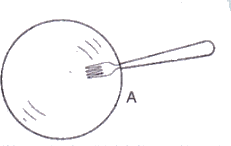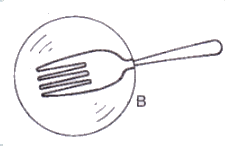Solution : (i) Concave lens because of negative magnification.
(ii) Convex lens because of positive magnification.

Question 25: What kind of lens can form :
(a) an inverted magnified image ?
(b) an erect magnified image ?
(c) an inverted diminished image ?
(d) an erect diminished image ?

Solution : (a) Convex lens.
(b) Convex lens.
(c) Convex lens.
(d) Concave lens.

Lakhmir Singh Physics Class 10 Solutions Page No:255

Question 1: The lens A produces a magnification of, – 0.6 whereas lens B produces a magnification of + 0.6.
(a) What is the nature of lens A ?
(b) What is the nature of lens B ?

Solution : (a) Convex lens (since image is real, inverted and diminished).
(b) Concave lens (since image is virtual, erect and diminished).

Question 2: A 50 cm tall object is at a very large distance from a diverging lens. A virtual, erect and diminished image of the object is formed at a distance of 20 cm in front of the lens. How much is the focal length of the lens ?

Solution : When an object is placed at a very large distance from a diverging lens, then image is formed at the focus of the lens.
Therefore, the focal length of the lens is 20 cm.

Page No:256

Question 3: An object is placed at a distance of 4 cm from a concave lens of focal length 12 cm. Find the position and nature of the image.

Solution :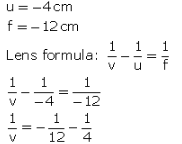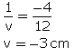Image is formed 3 cm infrcnt of the concave lens.

Image is virtual and erect.

Question 4: A concave lens of focal length 15 cm forms an image 10 cm from the lens. How far is the object placed from the lens ? Draw the ray-diagram.

Solution :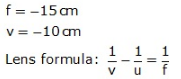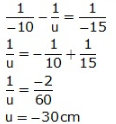Object is at 30 cm from the concave lens (on left side).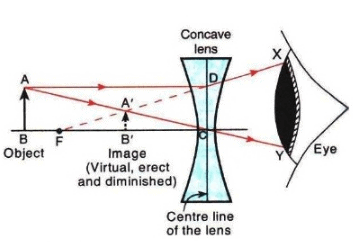Here, OB = 30cm
OF = 15cm
OB' = 10cm

Question 5: An object 60 cm from a lens gives a virtual image at a distance of 20 cm in front of the lens. What is the focal length of the lens ? Is the lens converging or diverging ?

Solution :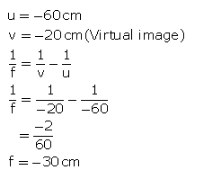The lens is diverging because the focal length is negative.

Question 6: A concave lens of 20 cm focal length forms an image 15 cm from the lens. Compute the object distance.

Solution :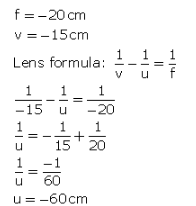Object distance is 60 cm towards the left of the lens.

Question 7: A concave lens has focal length 15 cm. At what distance should the object from the lens be placed so that it forms an image at 10 cm from the lens ? Also find the magnification produced by the lens.

Solution :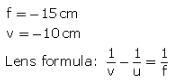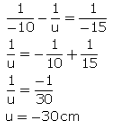Object should be placed at a distance of 60 cm on the left side of the lens.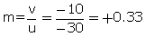Question 8: Calculate the image distance for an object of height 12 mm at a distance of 0.20 m from a concave lens of focal length 0.30 m, and state the nature and size of the image.

Solution :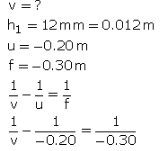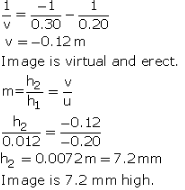Question 9: A concave lens has a focal length of 20 cm. At what distance from the lens a 5 cm tall object be placed so that it forms an image at 15 cm from the lens ? Also calculate the size of the image formed.

Solution :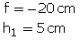v =-15cm (Concave lens forms virtual image)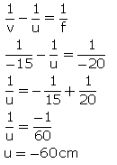Object should be placed 60 cm to the left of the lens.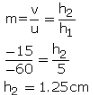Image formed is 1.25 cm high.

Question 10: An object is placed 20 cm from (a) a converging lens, and (b) a diverging lens, of focal length 15 cm. Calculate the image position and magnification in each case.

Solution :

u = - 20 cm
(a) f = 15cm (for converging lens)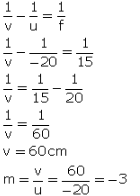(b) f = -15cm (for diverging lens)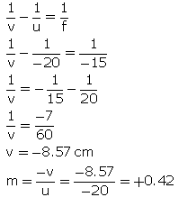Question 11: A 2.0 cm tall object is placed 40 cm from a diverging lens of focal length 15 cm. Find the position and size of the image.

Solution : h1 = 2 cm
u = - 40 cm
f = -15cm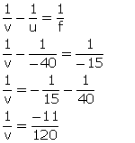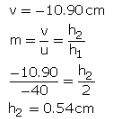Question 12: (a) Find the position and size of the virtual image formed when an object 2 cm tall is placed 20 cm from :
(i) a diverging lens of focal length 40 cm.
(ii) a converging lens of focal length 40 cm.
(b) Draw labelled ray diagrams to show the formation of images in cases (i) and (ii)above (The diagrams may not be according to scale).

Solution : h1 = 2 cm
u = - 20 cm
f = -40cm (Diverging lens)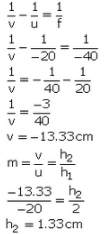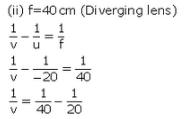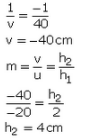(b) Formation of image in case (I):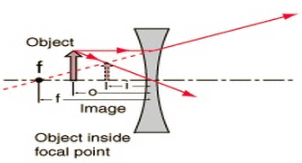Formation of image in case (ii):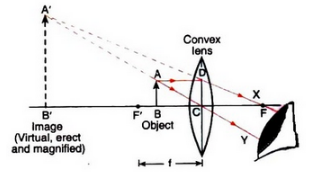Question 13: (a) A small object is placed 150 mm away from a diverging lens of focal length 100 mm.
(i) Copy the figure below and draw rays to show how an image is formed by the lens.
(ii) Calculate the distance of the image from the lens by using the lens formula.
(b) The diverging lens in part (a) is replaced by a converging lens also of focal length 100 mm. The object remains in the same position and an image is formed by the converging lens. Compare two properties of this image with those of the image formed by the diverging lens in part (a).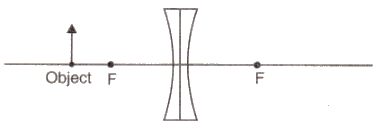Solution :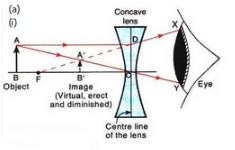Here, OB = 150 mm
OF = 100 mm

(ii) u = -150 mmm
f = -100 mm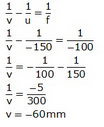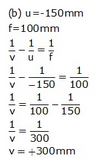The image formed by converging lens is real, inverted and magnified (2 times). It is formed behind the converging lens. On the other hand, the image formed by diverging lens is virtual, erect and diminished. It is formed in front of the diverging lens.

Page No:257

Question 18: A camera fitted with a lens of focal length 50 mm is being used to photograph a flower that is 5 cm in diameter. The flower is placed 20 cm in front of the camera lens.
(a) At what distance from the film should the lens be adjusted to obtain a sharp image of the flower ?
(b) What would be the diameter of the image of the flower on the film ?
(c) What is the nature of camera lens ?

Solution :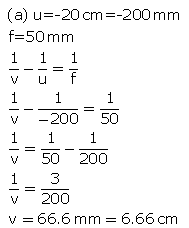The film should be at a distance of 6.66cm behind the camera lens,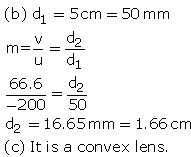Question 19: An object is 2 m from a lens which forms an erect image one-fourth (exactly) the size of the object. Determine the focal length of the lens. What type of lens is this ?

Solution :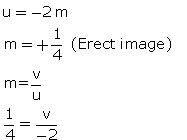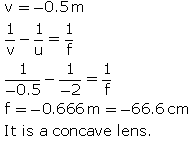Question 20: An image formed on a screen is three times the size of the object. The object and screen are 80 cm apart when the image is sharply focussed.
(a) State which type of lens is used.
(b) Calculate focal length of the lens.

Solution : (a) Since the image is formed on a screen, it must be a real image.

Hence, the lens should be a convex lens.

(b) m = -3 (Real and inverted image)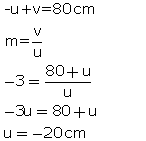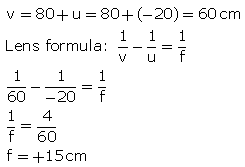Page No:261

Question 1: The lens A has a focal length of 25 cm whereas another lens B has a focal length of 60 cm. Giving reason state, which lens has more power : A or B

Solution : Lens A has more power because of its shorter focal length.

Question 2: Which causes more bending (or more refraction) of light rays passing through it: a convex lens of long focal length or a convex lens of short focal length ?

Solution : Convex lens of short focal length causes more bending of light rays passing through it.

Question 3: Name the physical quantity whose unit is dioptre.

Solution : Power of a lens.

Question 4: Define 1 dioptre power of a lens.

Solution : 1 diopter is the power of a lens whose focal length is 1 metre.

Question 5: Which typie of lens has (a) a positive power, and (b) a negative power ?

Solution : a) Positive power – Convex lens.
b) Negative power – Concave lens.

Question 6: Which of the two has a greater power : a lens of short focal length or a lens of large focal length ?

Solution : Lens of short focal length.

Question 7: How is the power of a lens related to its focal length ?

Solution : Power of lens is reciprocal of its focal length in metres.

Question 8: Which has more power : a thick convex lens or a thin convex lens, made of the same glass ? Give reason for your choice.

Solution : Thick convex lens has more power because of its shorter focal length.

Question 9: The focal length of a convex lens is 25 cm. What is its power ?

Solution : f = 25 cm = 0.25 cm.
P = 1/f = 1/0.25 = +4 D.

Question 10: What is the power of a convex lens of focal length 0.5 m ?

Solution : f = 0.5 m
P = 1/f = 1/0.5 = +2 D.

Question 11: A converging lens has a focal length of 50 mm. What is the power of the lens ?

Solution : f = 50 mm = 0.05 m
P = 1/f = 1/0.05 = +20 D.

Question 12: What is the power of a convex lens whose focal length is 80 cm ?

Solution : f = 80 cm = 0.8 m.
P = 1/f = 1/0.8 = +1.25 D.

Question 13: A diverging lens has a focal length of 3 cm. Calculate the power.

Solution : Here, f = -3 cm = -0.03 m (Diverging lens)
P = 1/f = 1/(-0.03) = -33.33 D.

Question 14: The power of a lens is + 0.2 D. Calculate its focal length.

Solution : P = +0.2 D.
P = 1/f.
f = 1/P = 1/0.2 = +5 m.

Question 15: The power of a lens is, – 2D. What is its focal length ?

Solution : P = -2 D.
P = 1/f.
f = 1/P = 1/(-2) = -0.5 m = -50 cm.

Question 16: What is the nature of a lens having a power of + 0.5 D ?

Solution : Convex lens.

Question 17: What is the nature of a lens whose power is, – 4 D ?

Solution : Concave lens.

Question 18: The optician’s prescription for a spectacle lens is marked + 0.5 D. What is the :
(a) nature of spectacle lens ?
(b) focal length of spectacle lens ?

Solution : (a) Convex lens
(b) P = +0.5 D.
P = 1/f.
f = 1/P = 1/0.5 = 2 m.

Question 19: A doctor has prescribed a corrective lens of power, -1.5 D. Find the focal length of the lens. Is the prescribed lens diverging or converging ?

Solution : P = -1.5 D
P = 1/f.
f = 1/P = 1/(-0.5) = -0.66 m = -66.6 m.
Since focal length is negative, it is a diverging lens.

Page No:262

Question 20: A lens has a focal length of, -10 cm. What is the power of the lens and what is its nature ?

Solution : f = -10 cm = -0.1 m
P = 1/f = 1/(-0.1) = -10 D
It is a concave lens.

Question 21: The focal length of a lens is +150 mm. What kind of lens is it and what is its power ?

Solution : f = +150 mm = +0.15 m
It is a convex lens since its focal length is positive.
P = 1/f = 1/0.15 = +6.66 D

Question 22: Fill in the following blanks with suitable words :
(a) The reciprocal of the focal length in metres gives you the……….. of the lens, which is measured in
(b) For converging lenses, the power is……… while for diverging lenses, the power is………….

Solution : (a) power, dioptres.
(b) positive, negative.

Question 23: An object of height 4 cm is placed at a distance of 15 cm in front of a concave lens of power, -10 dioptres. Find the size of the image.

Solution :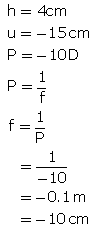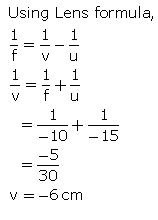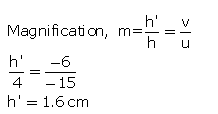Question 24: An object of height 4.25 mm is placed at a distance of 10 cm from a convex lens of power +5 D. Find (i) focal length of the lens, and (ii) size of the image.

Solution :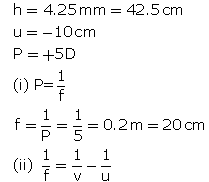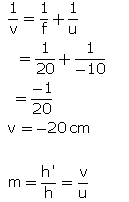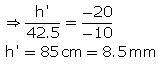Question 25: A convex lens of power 5 D and a concave lens of power 7.5 D are placed in contact with each other. What is the :
(a) power of this combination of lenses ?
(b) focal length of this combination of lenses ?

Solution :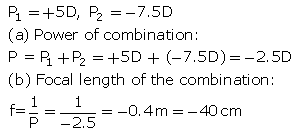Question 26: A convex lens of focal length 25 cm and a concave lens of focal length 10 cm are placed in close contact with one another.
(a) What is the power of this combination ?
(b) What is the focal length of this combination ?
(c) Is this combination converging or diverging ?

Solution :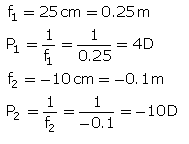(a) Power of the combination: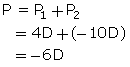(b) Focal length of the combination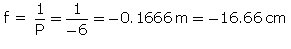(c) The combination has negative focal length, so it is diverging.

Question 27: The power of a combination of two lenses X and Y is 5 D. If the focal length of lens X be 15 cm :
(a) calculate the focal length of lens Y.
(b) state the nature of lens Y.

Solution :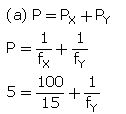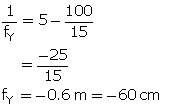(b) Lens Y is a concave lens since it has negative focal length.

Question 28: Two lenses A and B have focal lengths of + 20 cm and, -10 cm, respectively.
(a) What is the nature of lens A and lens B ?
(b) What is the power of lens A and lens B ?
(c) What is the power of combination if lenses A and B are held close together ?

Solution :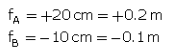(a) Lens A is a convex lens (positive focal length) and lens B is a concave lens (negative focal length).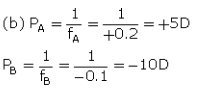(c) Power of combination
P = PA +PB = + 5D + (-10D) = -5D

Question 29: (a) What do you understand by the power of a lens ? Name one factor on which the power of a lens depends.
(b) What is the unit of power of a lens ? Define the unit of power of a lens.
(c) A combination of lenses for a camera contains two converging lenses of focal lengths 20 cm and 40 cm and a diverging lens of focal length 50 cm. Find the power and focal length of the combination.

Solution : (a) Power of a lens is a measure of the degree of convergence or divergence of light rays falling in it.

Power of a lens depends on its focal length.

(b) Unit of power of a lens is dioptre.

One dioptre is the power of a lens whose focal length is 1 metre.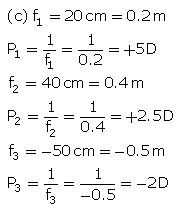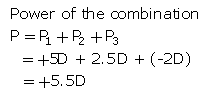Focal length orthr combination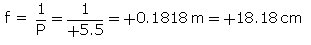Question 30: (a) Two lenses A and B have power of (i) + 2 D and (ii) – 4 D respectively. What is the nature and focal length of each lens ?
(b) An object is placed at a distance of 100 cm from each of the above lenses A and Calculate (i) image distance, and (ii) magnification, in each of the two cases.

Solution :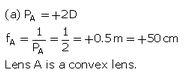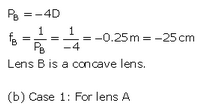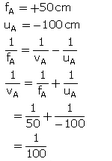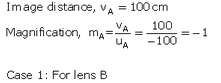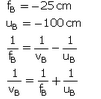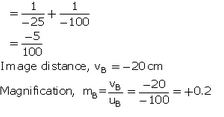Page No:263

Question 39: The optical prescription for a pair of spectacles is :
Right eye : – 3.50 D Left eye : – 4.00 D
(a) Are these lenses thinner at the middle or at the edges ?
(b) Which lens has a greater focal length ?
(c) Which is the weaker eye ?

Solution : (a) These lenses have negative powers and hence negative focal lengths, so they are concave lenses.
Concave lenses are thinner in the middle.
(b) Lens of lower power has greater focal length.
So, -3.50 D lens has greater focal length.
(c) Left eye is the weaker one because it needs a lens of greater power for its correction.

Question 40: A person got his eyes tested by an optician. The prescription for the spectacle lenses to be made reads :
Left eye : + 2.50 D Right eye : + 2.00 D
(a) State whether these lenses are thicker in the middle or at the edges.
(b) Which lens bends the light rays more strongly ?
(c) State whether these spectacle lenses will converge light rays or diverge light rays.

Solution : (a) These lenses have positive powers and hence positive focal lengths, so they are convex lenses.
Convex lenses are thicker in the middle.
(b) Lens of greater power bends light rays more quickly.
So, +2.50 D lens bends light rays more quickly.
(c) These spectacle lenses will converge the light rays because these are convex lenses.

Offer running on EduRev: Apply code STAYHOME200 to get INR 200 off on our premium plan EduRev Infinity!

61 docs

,

,

,

,

,

,

,

,

,

,

,

,

,

,

,

,

,

,

,

,

,

,

,

,

;